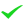# Machine Learning & Deep Learning Essentials with Spark and TensorFlow

Apache Spark, a significant component in the Hadoop Ecosystem, is a cluster computing engine used in Big Data. Building on top of the Hadoop YARN and HDFS ecosystem, offers order-of-magnitude faster processing for many in-memory computing tasks compared to Map/Reduce. It can be programmed in Java, Scala, Python, and R - the favorite languages of Data Scientists - along with SQL-based front ends. The first part of the course teaches performing Machine Learning at Scale using the popular Apache Spark framework. This course is intended for data scientists and software engineers, and assumes attendees have little or no previous experience with Machine Learning. This course explores popular machine learning algorithms from the ground up. Students will explore Apache Spark essentials, core machine learning concepts, regressions, classifications, clustering and more.

## Course Objectives

This “skills-centric” course is about 50% hands-on lab and 50% lecture, with extensive practical exercises designed to reinforce fundamental skills, concepts and best practices taught throughout the course.  Throughout the program, working in a hands-on learning environment guided by our expert instructor, students will

• Learn popular machine learning algorithms, their applicability, and limitations
• Practice the application of these methods in the Spark machine learning environment
• Learn practical use cases and limitations of algorithms
• Will explore not just the related APIs, but will also learn the theory behind them
• Work with real world datasets from Uber, Netflix, Walmart, Prosper, etc.

## Course Prerequisites

This is an intermediate level course, geared for Data Scientists, Data Analysts and Developers new to Machine Learning, Spark and TensorFlow.

Pre-Requisites:  Students should have attended or have incoming skills equivalent to those in this course:

• Strong basic Python Skills.  Attendees without Python background may view labs as follow along exercises or team with others to complete them.
• Good foundational mathematics in Linear Algebra and Probability
• Basic Linux skills, including familiarity with command-line options such as ls, cd, cp, and su

## Course Outline

Part 1: Introduction to Machine Learning

Machine Learning (ML) Overview

• Machine Learning landscape
• Machine Learning applications
• Understanding ML algorithms & models

ML in Python and Spark

• Spark ML Overview
• Introduction to Jupyter notebooks
• Lab: Working with Jupyter + Python + Spark
• Lab: Spark ML utilities

Machine Learning Concepts

• Statistics Primer
• Covariance, Correlation, Covariance Matrix
• Errors, Residuals
• Overfitting / Underfitting
• Cross-validation, bootstrapping
• Confusion Matrix
• ROC curve, Area Under Curve (AUC)
• Lab: Basic stats

Feature Engineering (FE)

• Preparing data for ML
• Extracting features, enhancing data
• Data cleanup
• Visualizing Data
• Lab: data cleanup
• Lab: visualizing data

Linear Regression

• Simple Linear Regression
• Multiple Linear Regression
• Running LR
• Evaluating LR model performance
• Lab
• Use case: House price estimates

Logistic Regression

• Understanding Logistic Regression
• Calculating Logistic Regression
• Evaluating model performance
• Lab: Use case: credit card application, college admissions

Classification: SVM (Supervised Vector Machines)

• SVM concepts and theory
• SVM with kernel
• Lab: Use case: Customer churn data

Classification: Decision Trees & Random Forests

• Theory behind trees
• Classification and Regression Trees (CART)
• Random Forest concepts
• Labs: Use case: predicting loan defaults, estimating election contributions

Classification: Naive Bayes

• Theory
• Lab
• Use case: spam filtering

Clustering (K-Means)

• Theory behind K-Means
• Running K-Means algorithm
• Estimating the performance
• Lab: Use case: grouping cars data, grouping shopping data

Principal Component Analysis (PCA)

• Understanding PCA concepts
• PCA applications
• Running a PCA algorithm
• Evaluating results
• Lab: Use case: analyzing retail shopping data

Recommendations (Collaborative filtering)

• Recommender systems overview
• Collaborative Filtering concepts
• Lab: Use case: movie recommendations, music recommendations

Performance

• Best practices for scaling and optimizing Apache Spark
• Memory caching
• Testing and validation

Part Two: Introduction to Deep Learning with TensorFlow

Introducing TensorFlow

• TensorFlow intro
• TensorFlow Features
• TensorFlow Versions
• GPU and TPU scalability
• Lab: Setting up and Running TensorFlow

The Tensor: The Basic Unit of TensorFlow

• Introducing Tensors
• TensorFlow Execution Model

Single Layer Linear Perceptron Classifier with TensorFlow

• Introducing Perceptrons
• Linear Separability and Xor Problem
• Activation Functions
• Softmax output
• Backpropagation, loss functions, and Gradient Descent
• Lab: Single-Layer Perceptron in TensorFlow

Hidden Layers: Intro to Deep Learning

• Hidden Layers as a solution to XOR problem
• Distributed Training with TensorFlow
• Vanishing Gradient Problem and ReLU
• Loss Functions
• Lab: Feedforward Neural Network Classifier in TensorFlow

High level TensorFlow: tf.learn

• Using high level TensorFlow
• Developing a model with tf.learn
• Lab: Developing a tf.learn model

Convolutional Neural Networks in TensorFlow

• Introducing CNNs
• CNNs in TensorFlow
• Lab : CNN apps

Introducing Keras

• What is Keras?
• Using Keras with a TensorFlow Backend
• Lab: Example with a Keras

Recurrent Neural Networks in TensorFlow

• Introducing RNNs
• RNNs in TensorFlow
• Lab: RNN

Long Short-Term Memory (LSTM) in TensorFlow

• Introducing RNNs
• RNNs in TensorFlow
• Lab: RNN

Conclusion

• Summarize features and advantages of TensorFlow
• Summarize Deep Learning and How TensorFlow can help
• Next steps
Course Dates Course Times (EST) Delivery Mode GTR
8/1/2022 - 8/5/2022 10:00 AM - 6:00 PM Virtual
10/3/2022 - 10/7/2022 10:00 AM - 6:00 PM Virtual12/5/2022 - 12/9/2022 10:00 AM - 6:00 PM Virtual